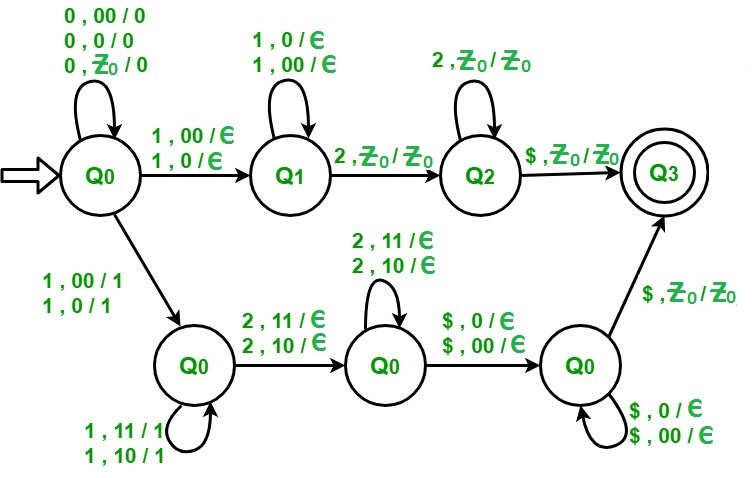GFG App
Open AppBrowser
Continue

# NPDA for L = {0i1j2k | i==j or j==k ; i , j , k >= 1}

Prerequisite – Pushdown automata, Pushdown automata acceptance by final state
The language L = {0i1j2k | i==j or j==k ; i , j , k >= 1} tells that every string of ‘0’, ‘1’ and ‘2’ have certain number of 0’s, then certain number of 1’s and then certain number of 2’s. The condition is that count of each of these 3 symbols should be atleast 1. Two important conditions for this language are that either count of 0 should be equal to count of 1 OR count of 1 should be equal to count of 2. Assume that string is ending with ‘\$’.

Examples:

```Input: 0 0 0 1 1 1 2 2 2 2 2
Here 0's = 3, 1's  = 3 so i = j, 2's = 5
Output: Accepted

Input: 0 0 1 1 1 2 2 2
Here 0's = 2, 1's = 3, 2's = 3 so j = k
Output: Accepted

Input : 0 0 1 1 1 2 2 2 2
Here 0's = 2, 1's = 3, 2's = 4
Output: Not accepted ```

There are 2 approaches for the solution. First is for i==j and second is for j==k. These are:

Steps for i == j :

1. Input all 0’s in the stack
2. When we get 1 as input pop a 0 from stack and goto next state.
3. If input is 1 then pop 0 from stack.
4. If stack becomes empty (i.e., every 0 corresponding to a 1 has been popped so i = j) and input is 2 then ignore it and goto next state.
5. If input is 2 then ignore it . If input is finished and \$ is received then goto final state.

Steps for j == k :

1. Input all 0’s in the stack
2. When we get 1 as input push it onto stack and goto next state.
3. If input is 1 then push it onto stack.
4. If input is 2 pop a 1 from stack and goto next state.
5. If input is 2 then pop 1 from stack. If input is finished and \$ is received then pop a 0 from stack.
6. Pop all remaining 0’s from the stack. If stack becomes empty then goto final state .My Personal Notes arrow_drop_up